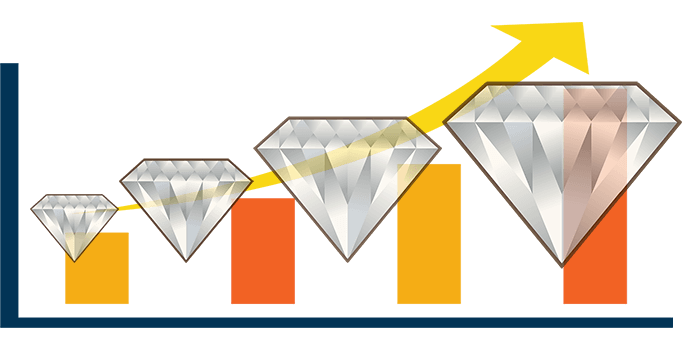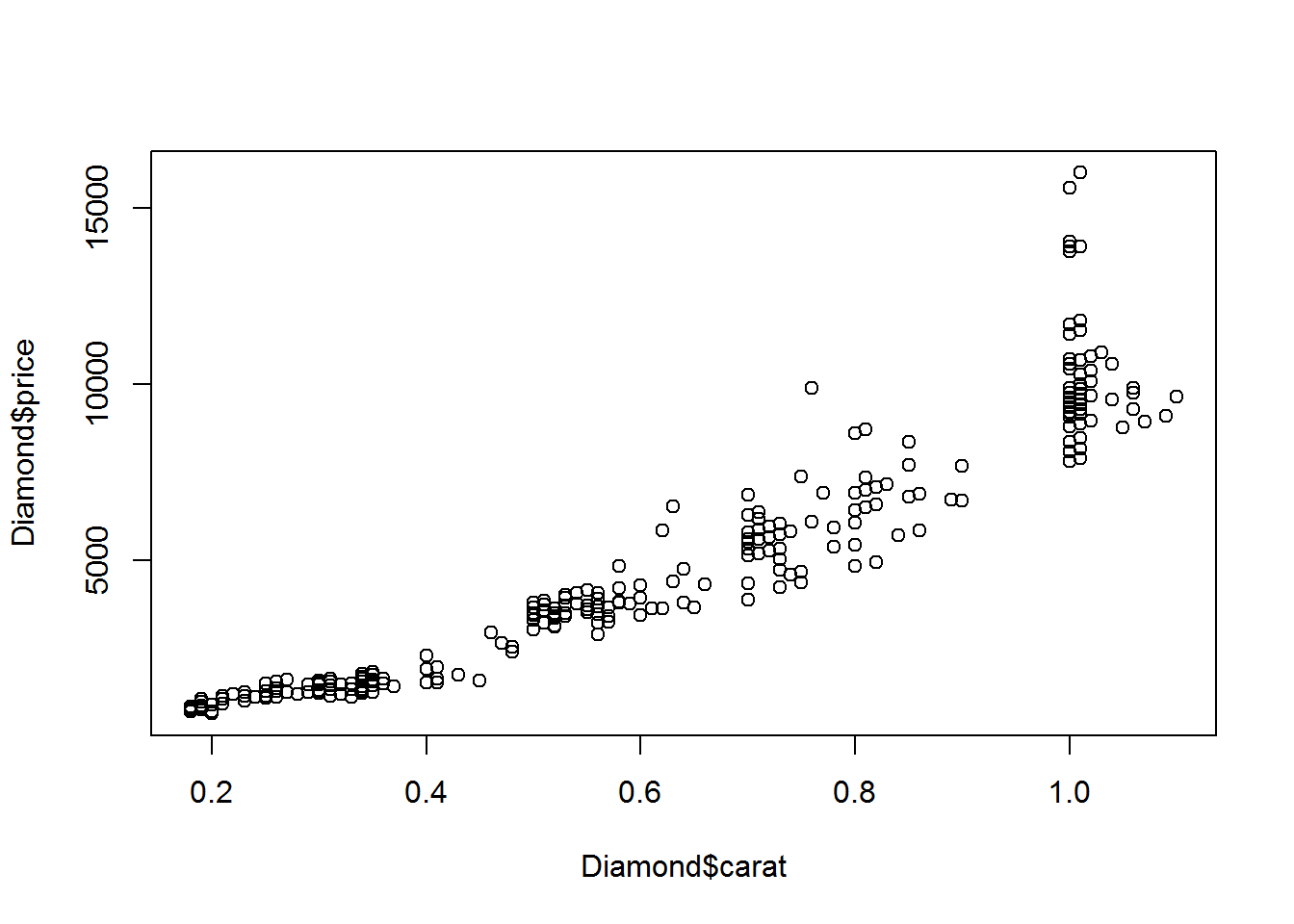# Case Study: Does the Size of Diamonds Affect Its Price?

If you have ever shopped for a diamond you already know the answer to the question this case study is posing. Try to buy a four carat diamond for $300 and the jeweler will first laugh at you, then throw you out of the store physically. He will tell you never to come back. Conversely,$300 could get you a really small diamond. But, it won’t come close to the four carats you were trying to buy.People familiar with how diamonds are priced will shout out that the size of the diamond (measured in carats) isn’t the only factor. In general, you must consider four factors (the four C’s). These are carat, color, cut, and clarity.

I choose this dataset on purpose to illutrate that there is more to data science than running a few simple models and getting complex questions answered. Diamonds are a complex product.

But, for the purposes of running through a simple linear regression, using just price and carat will suffice. t won’t be perfect, and you shouldn’t use the model as a means to price diamonds. Besides, it’s an old dataset, so the prices have likely changed at this point.

The relationship between carat and price can be be determined roughly. You already know that a larger carat will lead to a higher price all things being equal. The regression will give us that rough estimation we are looking for with the added bonus of keeping this case study as simple as possible.

This tutorial assumes you have a basic understanding of R programming and that you have knowledge of the concept of linear regression. If you need to learn about either of these concepts, I suggest you take a look at the this resource, which can learn both along with several other concepts.

## Step 1 – Load the Diamond dataset and print the first several rows.

  Diamond <- read.csv("https://vincentarelbundock.github.io/Rdatasets/csv/Ecdat/Diamond.csv")
head(Diamond)
##   X carat colour clarity certification price
## 1 1  0.30      D     VS2           GIA  1302
## 2 2  0.30      E     VS1           GIA  1510
## 3 3  0.30      G    VVS1           GIA  1510
## 4 4  0.30      G     VS1           GIA  1260
## 5 5  0.31      D     VS1           GIA  1641
## 6 6  0.31      E     VS1           GIA  1555

## Step 1 – Check the structure of the dataset.

  str(Diamond)
## 'data.frame':    308 obs. of  6 variables:
##  $X : int 1 2 3 4 5 6 7 8 9 10 ... ##$ carat        : num  0.3 0.3 0.3 0.3 0.31 0.31 0.31 0.31 0.31 0.31 ...
##  $colour : Factor w/ 6 levels "D","E","F","G",..: 1 2 4 4 1 2 3 4 5 6 ... ##$ clarity      : Factor w/ 5 levels "IF","VS1","VS2",..: 3 2 4 2 2 2 2 5 3 2 ...
##  $certification: Factor w/ 3 levels "GIA","HRD","IGI": 1 1 1 1 1 1 1 1 1 1 ... ##$ price        : int  1302 1510 1510 1260 1641 1555 1427 1427 1126 1126 ...

I use the str() command for every database that I am working. It gives you a snapshap of your data structure as well as some example data for each of the items. You should get in the habit of using it, too! .

## Step 2 – Plot the data.

  plot(Diamond$carat, Diamond$price)By creating a scatter plot, you can see immediately whether there exists a relationship between the two variables you are working with.

### Is plotting the data necessary?

Some peole may choose not to plot the data in the beginning. I believe this is a mistake. Plotting will help you get a birdseye view of your data. You can immediately notice any relationships or patterns and you can view any outliers that may appear in the data.

In most cases, the processing cost of running these plots are negligible. If you can get away with it, run the plot as part of your exploratory analysis.

## Step 3 – run a simple linear regression model

We want to run the regression model using weight as a function of height. That means our formula will contain weight as the response variable (dependent) and height as the explainer variable (independent).

  model <- lm(price ~ carat, Diamond)

Note: There is an instruction at the end that end of this tutorial that suggests change the price ~ caret to price ~ . The following is how it would look:

model <- lm(price ~ ., Diamond) The period (.) simply indicates all other variables of the dataset.

End of Note

Once you have your model, always run a summary on it.

  summary(model)
##
## Call:
## lm(formula = price ~ carat, data = Diamond)
##
## Residuals:
##     Min      1Q  Median      3Q     Max
## -2264.7  -604.3  -116.1   435.1  6591.5
##
## Coefficients:
##             Estimate Std. Error t value Pr(>|t|)
## (Intercept)  -2298.4      158.5  -14.50   <2e-16 ***
## carat        11598.9      230.1   50.41   <2e-16 ***
## ---
## Signif. codes:  0 '***' 0.001 '**' 0.01 '*' 0.05 '.' 0.1 ' ' 1
##
## Residual standard error: 1118 on 306 degrees of freedom
## Multiple R-squared:  0.8925, Adjusted R-squared:  0.8922
## F-statistic:  2541 on 1 and 306 DF,  p-value: < 2.2e-16

When you run the summary of the model, you can see what your coefficients are. More importantly, you will see which of the explainer variables are important to the model. Since this is a simple linear regression, there will only be one explainer variable and that will be carat.

In the coefficients section, you will notice a column Pr(|t|). This indicates the whether that coefficient is statistically significant or not. The threshold for what is statistically significant is up to the researcher (you). The most most common value for this is 5% (0.05). However, you could require a 10% or 1%. It could be whatever you want it to be (or whoever is paying says it should be!)

To interpret this value, let’s assume a 5% significance level. If the last column is lower than 0.05, then you have some confidence that the coefficient in question is statistically significant. In our case, carat is well below 0.05, so it is reasonable to assume that this coefficient will have an effect on the dependent variable.

In the last section of the summary results, you’ll se the item called Multiple R-Squared. In our example, it is close to .90, which is quite hight. This suggests that this independent variable can explain about 90% of this relationship. In other words, it’s a good fit.

## Conclusion

The purpose of this exercise was to get you familiar with what it takes to run a simple linear regression. It is clearly an oversimplification. If you were to run a multivariate regression, you would see the other coefficients that may or may not affect the relationship between carat and price.

As an exercise, you could change the example quite easily to run a multivariate regression. Go through all the steps again. But, when you get to the regression call, simply replace lm(price ~ carat, Diamond) with lm(price ~ ., Diamond). Take a look at the results and see how that affects the model.

Don’t blow a gasket in your brain trying to figure out all of the coefficients if you decide to try the multivariate approach. Just understand that there is more to modeling than running a simple regression in most cases.

## Code for this Case Study

For convenience, I have included the full code in the following section so you can simply copy and paste into your R session. It should run without any trouble.

#Load the data and display the first few rows

# Take a look at the structure of the data
str(Diamond)

# Plot the data to see what relationship exists
plot(Diamond$carat, Diamond$price)

# Run the simple linear regression
model <- lm(price ~ carat, Diamond)

# Run a summary on the model to analyze the results
summary(model)

Enjoy!